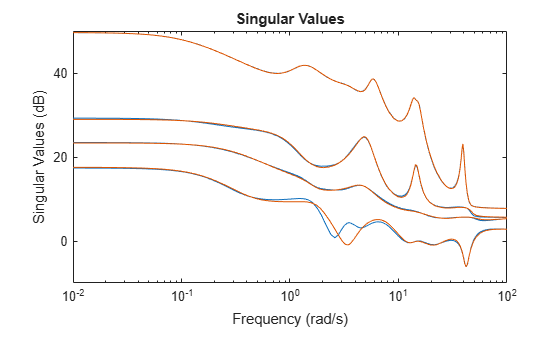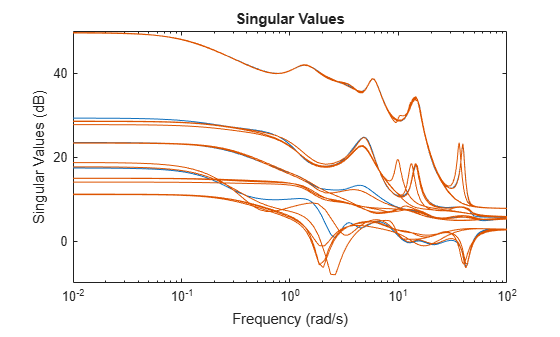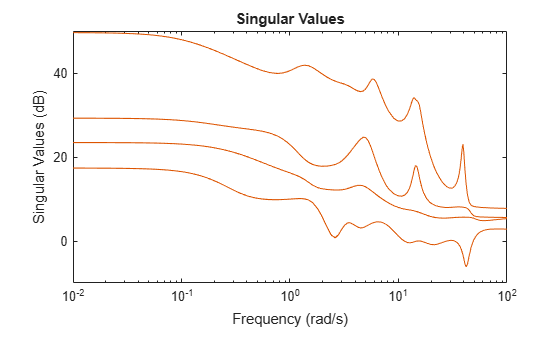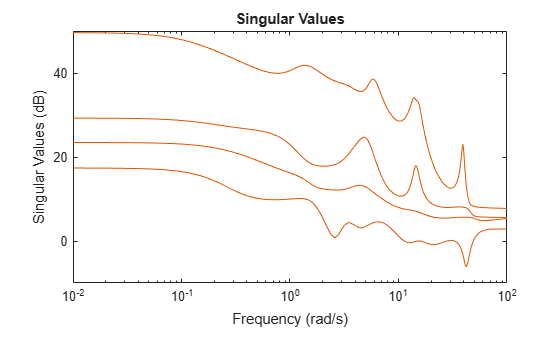# reduce

## Syntax

```GRED = reduce(G)
GRED = reduce(G,order)
[GRED,redinfo] = reduce(G,'key1','value1',...)
[GRED,redinfo] = reduce(G,order,'key1','value1',...)
```

## Description

`reduce ` returns a reduced order model `GRED` of `G` and a struct array `redinfo` containing the error bound of the reduced model, Hankel singular values of the original system and some other relevant model reduction information.

An error bound is a measure of how close `GRED` is to `G` and is computed based on either additive error,`G-GRED`, multiplicative error,`G`–1`(G-GRED)`, or nugap error (ref.: `ncfmr`) ,,.

Hankel singular values of a stable system indicate the respective state energy of the system. Hence, reduced order can be directly determined by examining the system Hankel SV's. Model reduction routines, which based on Hankel singular values are grouped by their error bound types. In many cases, the additive error method `GRED=reduce(G,ORDER)` is adequate to provide a good reduced order model. But for systems with lightly damped poles and/or zeros, a multiplicative error method (namely, `GRED=reduce(G,ORDER,'ErrorType','mult')`) that minimizes the relative error between `G` and `GRED` tends to produce a better fit.

This table describes input arguments for `reduce`.

Argument

Description

`G`

LTI model to be reduced (without any other inputs will plot its Hankel singular values and prompt for reduced order).

`ORDER`

(Optional) Integer for the desired order of the reduced model, or optionally a vector packed with desired orders for batch runs.

A batch run of a serial of different reduced order models can be generated by specifying `order = x:y`, or a vector of integers. By default, all the anti-stable part of a physical system is kept, because from control stability point of view, getting rid of unstable state(s) is dangerous to model a system.

`'``MaxError``'` can be specified in the same fashion as an alternative for `'` `ORDER` `'` after an `'``ErrorType``'` is selected. In this case, reduced order will be determined when the sum of the tails of the Hankel SV's reaches the `'``MaxError``'`.

Argument

Value

Description

`'Algorithm'`

`'balance'`

`'schur'`

`'hankel'`

`'bst'`

`'ncf'`

Default for `'add'` (`balancmr`)

Option for `'add'` (`schurmr`)

Option for `'add'` (`hankelmr`)

Default for `'mult'` (`bstmr`)

Default for `'ncf'` (`ncfmr`)

`'ErrorType'`

`'add'`

`'mult'`

`'ncf'`

Multiplicative error at model output

NCF nugap error

`'MaxError'`

A real number or a vector of different errors

Reduce to achieve H error.

When present, `'``MaxError``'` overrides `ORDER` input.

`'Weights'`

`{Wout,Win}` cell array

Optimal 1x2 cell array of LTI weights `Wout` (output) and `Win` (input); default is both identity; used only with `'``ErrorType``'`, `'``add``'`. Weights must be invertible.

`'Display'`

`'``on``'` or `'``off``'`

Display Hankel singular plots (default `'``off``'`).

`'Order'`

Integer, vector or cell array

Order of reduced model. Use only if not specified as 2nd argument.

Weights on the original model input and/or output can make the model reduction algorithm focus on some frequency range of interests. But weights have to be stable, minimum phase and invertible.

This table describes output arguments.

Argument

Description

`GRED`

LTI reduced order model. Becomes multi-dimensional array when input is a serial of different model order array.

`REDINFO`

A STRUCT array with 3 fields:

• `REDINFO.ErrorBound`

• `REDINFO.StabSV`

• `REDINFO.UnstabSV`

For `'hankel'` algorithm, STRUCT array becomes:

• `REDINFO.ErrorBound`

• `REDINFO.StabSV`

• `REDINFO.UnstabSV`

• `REDINFO.Ganticausal`

For `'ncf'` option, STRUCT array becomes:

• `REDINFO.GL`

• `REDINFO.GR`

• `REDINFO.hsv`

`G` can be stable or unstable. `G` and `GRED` can be either continuous or discrete.

A successful model reduction with a well-conditioned original model `G` will ensure that the reduced model `GRED` satisfies the infinity norm error bound.

## Examples

collapse all

Given a continuous or discrete, stable or unstable system, `G`, create a set of reduced-order models based on your selections.

```rng(1234,'twister'); % For reproducibility G = rss(30,5,4);```

If you call `reduce` without specifying an order for the reduced model, the software displays a Hankel singular-value plot and prompts you to select an order.

If you specify a reduced-model order, `reduce` defaults to the `balancmr` algorithm for model reduction.

`[g1,redinfo1] = reduce(G,20);`

Specify other algorithms using the `Algorithm` argument. Use the `ErrorType` argument to specify whether the algorithm uses multiplicative or additive error, and the maximum permissible error in the reduced model.

```[g2,redinfo2] = reduce(G,[10:2:18],'Algorithm','schur'); [g3,redinfo3] = reduce(G,'ErrorType','mult','MaxError',[0.01 0.05]); [g4,redinfo4] = reduce(G,'ErrorType','add','Algorithm','hankel','MaxError',[0.01]); for i = 1:4 figure(i); eval(['sigma(G,g' num2str(i) ');']); end```## References

 K. Glover, “All Optimal Hankel Norm Approximation of Linear Multivariable Systems, and Their L- error Bounds,” Int. J. Control, vol. 39, no. 6, pp. 1145-1193, 1984.

 M. G. Safonov and R. Y. Chiang, “A Schur Method for Balanced Model Reduction,” IEEE Trans. on Automat. Contr., vol. AC-2, no. 7, July 1989, pp. 729-733.

 M. G. Safonov, R. Y. Chiang and D. J. N. Limebeer, “Optimal Hankel Model Reduction for Nonminimal Systems,” IEEE Trans. on Automat. Contr., vol. 35, No. 4, April, 1990, pp. 496-502.

 M. G. Safonov and R. Y. Chiang, “Model Reduction for Robust Control: A Schur Relative-Error Method,” International Journal of Adaptive Control and Signal Processing, vol. 2, pp. 259-272, 1988.

 K. Zhou, “Frequency weighted L[[BULLET]] error bounds,” Syst. Contr. Lett., Vol. 21, 115-125, 1993.